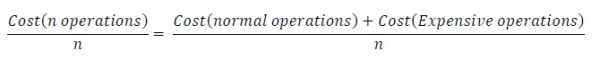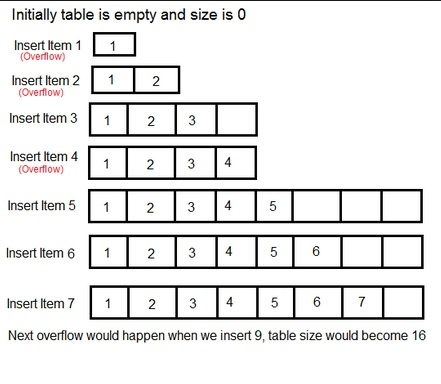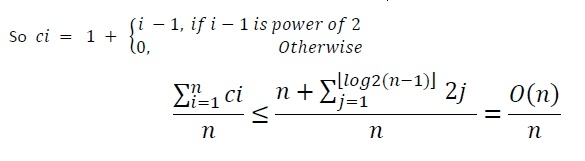# Amortized Complexity

Data StructureAlgorithmsAnalysis of Algorithms

## Amortize Analysis

This analysis is used when the occasional operation is very slow, but most of the operations which are executing very frequently are faster. In Data structures we need amortized analysis for Hash Tables, Disjoint Sets etc.

In the Hash-table, the most of the time the searching time complexity is O(1), but sometimes it executes O(n) operations. When we want to search or insert an element in a hash table for most of the cases it is constant time taking the task, but when a collision occurs, it needs O(n) times operations for collision resolution.

## Aggregate Method

The aggregate method is used to find the total cost. If we want to add a bunch of data, then we need to find the amortized cost by this formula.

For a sequence of n operations, the cost is −## Example on Amortized Analysis

For a dynamic array, items can be inserted at a given index in O(1) time. But if that index is not present in the array, it fails to perform the task in constant time. For that case, it initially doubles the size of the array then inserts the element if the index is present.For the dynamic array, let 𝑐𝑖 = cost of 𝑖𝑡ℎ insertion.